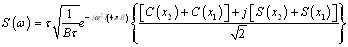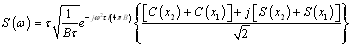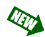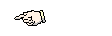Design of Multi-Frequency CW Radars by M. Jankiraman Published by SciTech Publishing Inc., August 2007

There is no software CD supplied with the book. This software can now be freely downloaded from the publisher's website at:
https://www.scitechpublishing.com/index.asp?PageAction=VIEWPROD&ProdID=70

Errata

List of Acronyms
For:
VFM    Vertical FM
VFM    V (Shaped) FM

1. Chapter 1

Section 1.2
For:
This section is based on the work done by Pace (From  © 2004, . . .
This section is based on the work done by Pace (From  © 2004, . . .

The error is shown in red.

2. Chapter 2

Section 2.3
Sub-section 2.3.4.2
Second para, last line:
For:
This method is also called fast convolution processing (FCP).
This method is called digital correlation.

Para entitled "Circular Correlation":
Add after last line:
This method is also called fast convolution processing (FCP).

The corrections are shown in red.

Sub-section 2.3.8
For (equation 2.23):3. Chapter 3

Section 3.2
Sub-section 3.2.1
Page 44, last para:
For:
In out case, it is at 0.5 Hz, since T = 2 second.
In our case, it is at 0.5 Hz, since T = 2 second.

The error is shown in red.

Sub-section 3.2.4
For:
Digital Coded Waveforms can be classified into the following major groups:

• Phase-coded waveforms. This class of waveforms can be further grouped into two major groups:
• Binary phase codes, like Barker codes .
• Polyphase codes like Frank codes .
• Frequency-coded waveforms like Costas codes .

Digital Coded Waveforms can be classified into the following major groups:

• Phase-coded waveforms. This class of waveforms can be further grouped into two major groups:
- Binary phase codes, like Barker codes .
- Polyphase codes like Frank codes .
• Frequency-coded waveforms like Costas codes .

There is a formatting error and is shown in red.

4. Chapter 4Section 4.1
Delete
We also studied an antitank missile, which uses continuous waveforms (CW) radar technology for reasons of low probability of intercept (LPI).

Add at end of para (Section 4.1):
Finally, we will study an antitank missile, which uses continuous waveforms (CW) radar technology for reasons of low probability of intercept (LPI).

Section 4.2
Sub-section 4.2.2.1
Page 58, first para, last line:
For:
We now examine the math behind this process with reference to FCP as shown in Figure 4-13.
We now examine the math behind this process with reference to FCP as shown in Figure 2-13.

The error is shown in red.

Page 59, Figure 4-3 (b): The figure title should read:
Two targets separated 10 m apart: Sweep bandwidth 50 MHz

The error is shown in red

Section 4.9
First line:
For:
Normally, the radar range equation like (1.22) gives us ....
Normally, the radar range equation like (1.9) gives us ....

Section 4.10
First line:
For:
In order to illustrate the problem, we utilize equation (1.27) to .....
In order to illustrate the problem, we utilize equation (1.13) to .....

Page 78, last line:
For:
Using equation (1.27),....
Using equation (1.13),....

The errors are shown in red.

Sub-section 4.10.1
Page 82, first line:
For:
Using results of example in Section 4.6 above, ....
Using results of example in Section 4.10 above, ....

Just below equation (4.63):
For:
Once again, using the example in Section 4.6, the left....
Once again, using the example in Section 4.10, the left....

The errors are shown in red.

Section 4.11
Sub-section 4.11.2
Delete:
(see Figure 4.18)

Sub-section 4.11.3
Delete:
(see Figure 4.20)

Page 88 (last line)
For:
The antenna uses an interesting Cassegrain configuration  with a scanned parabolic mirror as shown in Figure 4-27.
The antenna uses an interesting Cassegrain configuration  with a scanned parabolic mirror as shown in Figure 4-23.

The error is shown in red.

Page 89:
For:
It can be assumed that a single mechanical scan takes place in the 200 msec search time (see Figure 4-24).
It can be assumed that a single mechanical scan takes place in the 200 msec search time (see Figure 4-20).

The error is shown in red.

5. Chapter 6

Page 151, reference :
For:
1. Levanon, N., Radar Principles, John Wiley & Sons, New York, 1988.
1. Levanon, N. and Mozeson, E., Radar Signals, Wiley-Interscience, Hoboken, New Jersey, June 25, 2004.

6. Chapter 7

Section 7.5
Page 160, last line:
Delete:
...(see Appendix K)...

7. Chapter 8

Section 8.5.1
Page 191, first para,
For:
However, there are two broad classes of signals viz. frequency modulated such as LFM, VFM (this is basically up and down chirp juxtaposed next to each other, yielding a V shape giving rise to its name.....
However, there are two broad classes of signals viz. frequency modulated such as LFM, VFM (this is basically down followed by an up chirp juxtaposed next to each other, yielding a V shape giving rise to its name.....

The correction is shown in red.

Page 192, second last line:
For:
In view off the structure......
In view of the structure......

The error is shown in red.

Page 194, just above equation (8.2):
Delete:
In the Pandora radar, there is one more reason why we need to reject it.

Section 8.6.2
Page 200, last line:
For:
We can see the output from the combiner in Figure 8-15.
We can see the output from the combiner in Figure J-5 (Appendix J).

The error is shown in red.

Section 8.10
On page 211:
For:
Hence, we need to ensure proper channel isolation using steep baseband filters as discussed in Section 8.5.8.
Hence, we need to ensure proper channel isolation using steep baseband filters as discussed in Section 8.6.8.

The error is shown in red.

Section 8.11
Page 213, Fig 8.23 is actually Fig 8.22.

Page 219, reference :
For:
Levanon, N., Radar Principles, John Wiley & Sons, New York, 1988.
Levanon, N. and Mozeson, E., Radar Signals, Wiley-Interscience, Hoboken, New Jersey, June 25, 2004.

8. Chapter 9

Section 9.6.3
Para 1: For Figure 8.20 read Figure 8.21

Para 2: For Figure 8.21 read Figure 8.20

Section 9.8
Page 226, para 2:
For:
It can also be used in a pulsed mode as a signal source for pulsed radar.
It can also be used in pulsed mode as a signal source for pulse modulated radars.

The new phrase is shown in red.

9.Chapter 10

Caption for Figure 10-15:
For:
Experimental setup for mine detection using Pandora. (Courtesy IRCTR: Reprinted with permission.)
Experimental setup for mine detection using Pandora. Note the transmitter and receiver cabinets in the background. (Courtesy IRCTR: Reprinted with permission.)

The additional phrase is shown in red.

Section 10.7

First line:
For:
The Pandora type of multifrequency architecture lends itself not only for CW applications but also pulse radar applications. This includes both unmodulated as well as chirp pulse radars.
The Pandora type of multifrequency architecture lends itself not only for CW applications but also pulse modulated radar applications. This includes both phase modulated as well as chirp pulse radars.

The modified phrase is shown in red.

10. Appendix B

Section 1.3
Para 1:
For:
A 6-order Butterworth fits these requirements. This will be an active filter (see Figure B-2).
A 6-order Butterworth fits these requirements (see Figure B-2).

The modified phrase is shown in red.

11. Appendix E

Para 1, last line:
For:
This derivation is based on the paper by Stove .
This derivation is based on the paper by Stove  and is reproduced here with the permission of IEE.

The additional phrase is shown in red.

12. Appendix K

Page 343, 3rd line:
For:
To summarize, the following procedure are recommended:
To summarize, the following procedure is recommended:

The error is shown in red.

Note: If readers notice any more errors in the book, they are requested to intimate the author at mjankiraman@ieee.org. These will be gratefully received!

Readers are advised to check this site periodically for errors/updates.